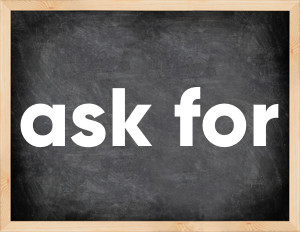The English verb 'ask for' is pronounced as [ɑːsk fɔː].
Related to: phrasal verb.

## Here are the past tense forms of the verb ask for

👉 Forms of verb ask for in future and past simple and past participle.
❓ What is the past tense of ask for.

## Ask for: Past, Present, and Participle Forms

Base Form Past Simple Past Participle

## What are the 2nd and 3rd forms of the verb ask for?

🎓 What are the past simple, future simple, present perfect, past perfect, and future perfect forms of the base form (infinitive) 'ask for'?

### Learn the three forms of the English verb 'ask for'

• the first form (V1) is 'ask for' used in present simple and future simple tenses.
• the second form (V2) is 'asked for' used in past simple tense.
• the third form (V3) is 'asked for' used in present perfect and past perfect tenses.

## What are the past tense and past participle of ask for?

The past tense and past participle of ask for are: ask for in past simple is asked for, and past participle is asked for.

### What is the past tense of ask for?

The past tense of the verb "ask for" is "asked for", and the past participle is "asked for".

### Verb Tenses

Future simple — ask for in future simple is ask for (will + V1).
Present Perfect — ask for in present perfect tense is asked for (have/has + V3).

### ask for regular or irregular verb?

👉 Is 'ask for' a regular or irregular verb? The verb 'ask for' is .

## Examples of Verb ask for in Sentences

•   The employees asked for a raise. (Past Simple)
•   He asked the cashier for two five-dollar bills instead of a 10-dollar bill. (Past Simple)
•   Ask for help (Present Simple)
•   Ask for price (Present Simple)
•   Ask for raise (Present Simple)
•   Ask for money (Present Simple)
•   Ask for pardon  (Present Simple)
•   Ask for leave (Present Simple)
•   Ask for help if necessary (Present Simple)